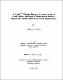## A H and 3C nuclear magnetic resonance study of renal, adrenal and hepatic metabolism in Meriones unguiculatus infected with Echinococcus multilocularisNuclear magnetic resonance (NMR) spectroscopy was utilized to test the hypothesis that infection with the cestode parasite Echinococcus multilocularis will result in metabolic alterations in the kidneys, adrenal glands and liver of the intermediate host Meriones unguiculatus. Kidneys from infected animals had lower concentrations of phosphatidylcholine (PTC), phosphatidylethanolamine (PTE), total lycerophospholipid (GPL), arachidonic acid (AA) and CH$\sb2$ in the unsaturated fatty acid (FA) moiety -CH=CH(CH$\sb2$CH=CH)$\rm\sb{n}$-. In addition, the ratios of both AA and CH$\sb2$ in -CH=CH(CH$\sb2$CH=CH)$\rm\sb{n}$- to total FA decreased in infected animals. Meanwhile, the adrenal glands from infected jirds had lower concentrations of cholesterol (CTL), PTE, total GPL, total FA, total triacylglycerol, linoleic acid, and the FA moieties -CH$\sb2$CH$\sb2$COO, -CH$\sb2$COO, -(C$\rm\sb2)\sb{n}$-, -CH=CH-, -CH$\sb2$CH=CH- and CH$\sb2$ in CH=CH(CH$\sb2$CH=CH)$\rm\sb{n}$-. The adrenal concentrations of AA, docosahexaenoic acid (DHA) and CTL esters were unchanged. The ratios of most FA moieties to total FA all decreased in infected animals, while that of DHA increased and that of CH$\sb2$ in -CH=CH(CH$\sb2$CH=CH)$\rm\sb{n}$- remained the same. The average FA chain length and degree of unsaturation were also lower. Perchloric acid (PCA) extracts of livers were analyzed following portal vein injections of an equimolar mixture of (1,2-$\rm\sp{13}C\sb2$) acetate and (3-$\sp{13}$C) lactate. The relative contributions of endogenous and exogenous substrates to the acetyl coenzyme A entering the TCA cycle differed significantly between infected and control groups. In uninfected animals, the relative proportion of acetyl-CoA derived from labeled lactate (F$\rm\sb{LL})$ was 0.18, that from labeled acetate (F$\rm\sb{LA})$ was 0.32 and that from endogenous, unlabeled sources (F$\rm\sb{U})$ was 0.50. The corresponding values from livers of infected animals were 0.27, 0.38 and 0.34, respectively. In addition, the ratio of F$\rm\sb{LA}$:F$\rm\sb{LL}$ was significantly smaller in the infected group, while the percentage of $\sp{13}$C defected at carbon-3 of glutamate was the same in both groups. (Abstract shortened by UMI.)